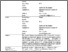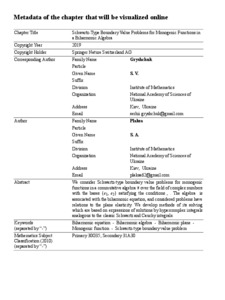Schwartz-Type Boundary Value Problems for Monogenic Functions in a Biharmonic Algebra

Плакса, С. А. and Грищук, С. В. (2019) Schwartz-Type Boundary Value Problems for Monogenic Functions in a Biharmonic Algebra. Springer Nature Switzerland AG.Preview
Text

Abstract

We consider Schwartz-type boundary value problems for monogenic functions in a commutative algebra over the field of complex numbers with the bases {e1, e2} satisfying the conditions , . The algebra is associated with the biharmonic equation, and considered problems have relations to the plane elasticity. We develop methods of its solving which are based on expressions of solutions by hypercomplex integrals analogous to the classic Schwartz and Cauchy integrals.

Item Type: Article Biharmonic equation - Biharmonic algebra - Biharmonic plane - Monogenic function - Schwartz-type boundary value problem Q Science > QA Mathematics > Mathematical Analysis Faculty of Physics and Mathematics > Department of Mathematical Analysis Ірина Ігорівна Таргонська 26 Feb 2019 09:35 26 Feb 2019 09:37 http://eprints.zu.edu.ua/id/eprint/28411View Item